Next: Why the causal wavelet Up: SPECTRAL FACTORIZATION Previous: The exponential of a

## Finding a causal wavelet from a prescribed spectrum

To find a causal wavelet from a prescribed spectrum, we will need to form the logarithm of the spectrum. Since a spectrum can easily vanish, and since the logarithm of zero is infinite, there is a pitfall. To prepare ourselves, we first examine the log spectra example given in Figure 10. On the infinite domain, the FT of a box function is a sinc whose zeros become minus infinities in the logarithm. On the discrete domain, exact zeros may occur or not. The transform of a triangle is a sinc squared, but since this triangle was imperfectly drawn (by me), its transform does not go identically to zero. The sinc function drops off as,and sinc squared drops off as.We confirm this on the logarithm plot: sinc squared drops off twice as much.

 logspec Figure 10 Log spectra of a box function and a triangle function.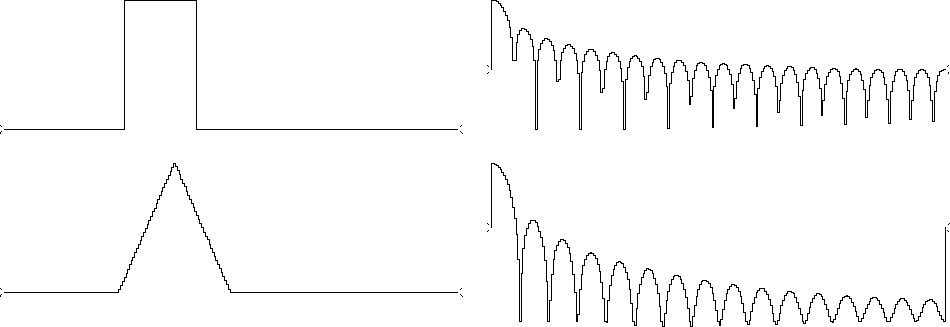Now for the task of going from a spectrum to a causal wavelet. Take as given the spectrum of the causal wavelet B(Z). This means that we are not given B(Z) itself, but we are given.Assuming no zeros in the spectrum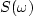,it is easy to find the log of the spectrum.The spectrum may be specified as autocorrelation coefficients or values on the unit circle. Thus,(18)
Given the spectrum S(Z) for each value on the unit circle, we could deduce the log spectrum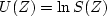at each point on

the unit circle: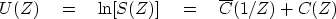(19)
This is the answer we have been looking for. Given U(Z) for all real values of, we could inverse transform to the time domain, obtaining the two-sided function.Setting to zero the coefficients at negative times eliminates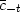, leaving just ct; hence C(Z). And we already know that the exponential of C(Z) gives B(Z) with a causal bt. This method is known as Kolmogoroff spectral factorization," after the mathematician who discovered it.

The program mpwave() below begins with a wavelet, forms its spectrum, and then calls kolmogoroff() to factor the spectrum. The program kolmogoroff() first takes the logarithm of the spectrum, then returns to the time domain and sets to zero the noncausal part. It returns to frequency, exponentiates, and returns to the time domain with a wavelet that will be proven to be minimum-phase.

subroutine mpwave( n, cx)       # minimum phase equivalent wavelet
integer i,         n            # input:  cx = any wavelet
complex cx(n)                   # output: cx = min phase wavelet
call ftu( 1., n, cx)            #               with same spectrum.
call scaleit( sqrt(1.*n), 2*n, cx)
do i= 1, n
cx(i) = cx(i) * conjg( cx(i))
call kolmogoroff( n, cx)
return; end


subroutine kolmogoroff( n, cx)  # Spectral factorization.
integer i,              n       # input:  cx = spectrum
complex cx(n)                   # output: cx = min phase wavelet
do i= 1, n
cx(i) = clog( cx(i) )
call ftu( -1., n, cx);          call scaleit( sqrt(1./n), 2*n, cx)
cx(1    ) = cx(1    ) / 2.
cx(1+n/2) = cx(1+n/2) / 2.
do i= 1+n/2+1, n
cx(i) = 0.
call ftu( +1., n, cx);          call scaleit( sqrt(1.*n), 2*n, cx)
do i= 1, n
cx(i) = cexp( cx(i))
call ftu( -1., n, cx);          call scaleit( sqrt(1./n), 2*n, cx)
return; end


Between the times when negative lags are set to zero and positive lags are left untouched are two points that are scaled by half. The overall scaling was chosen to preserve the scale of the input wavelet.

The first test I tried on this program was the input wavelet (1,2,0,0). The desired result is that the wavelet should time-reverse itself to (2,1,0,0). The actual result was (1.9536, 1.0837, 0.0464, -0.0837), imperfect because the four-point Fourier transform is a summation around the unit circle, whereas theoretically an integration is called for. Therefore, better results can be obtained by padding additional zeros after the input wavelet. Also, you might notice that the program is designed for complex-valued signals. As typical of Fourier transform with single-word precision, the imaginary parts were about 10-6 of the real parts instead of being precisely zero.

Some examples of spectral factorization are given in Figure 11.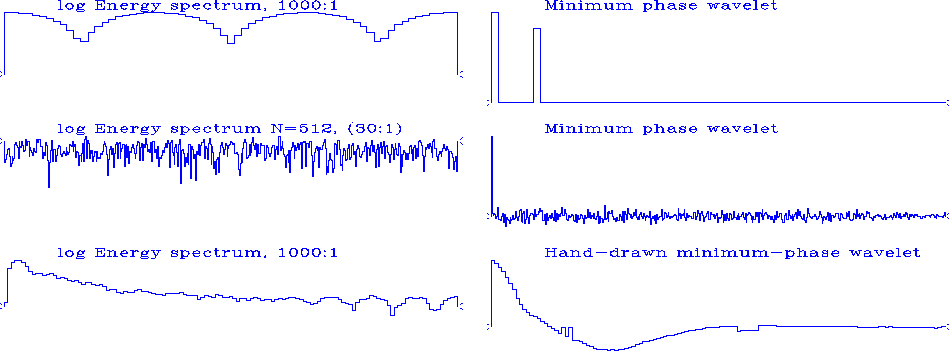example
Figure 11
Examples of log spectra and their associated minimum-phase wavelets.Next: Why the causal wavelet Up: SPECTRAL FACTORIZATION Previous: The exponential of a
Stanford Exploration Project
10/21/1998